# Convert Time to Hours – Excel & Google Sheets

This tutorial will demonstrate how to convert a time value to hours in Excel & Google Sheets.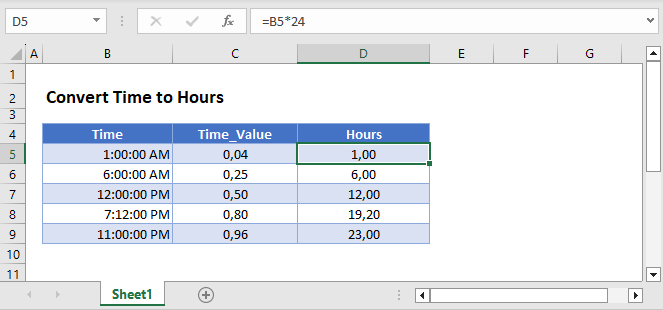## Convert Time to Hours – Excel

Excel stores time as a decimal value where each 1/24th represents one hour of a day. Understanding this, you can convert a time to hours by multiplying the time by 24:

``=B3*24``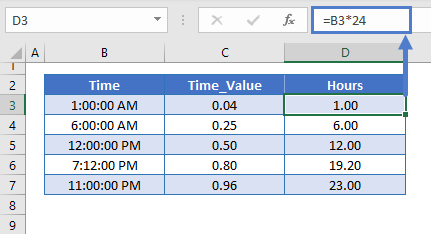### Format as Number

When you multiply time by 24, Excel may format the result as time (ex. h:mm):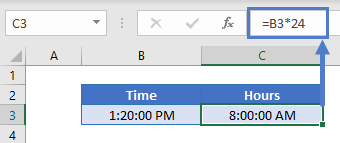To display the result as a regular number, change the formatting to General: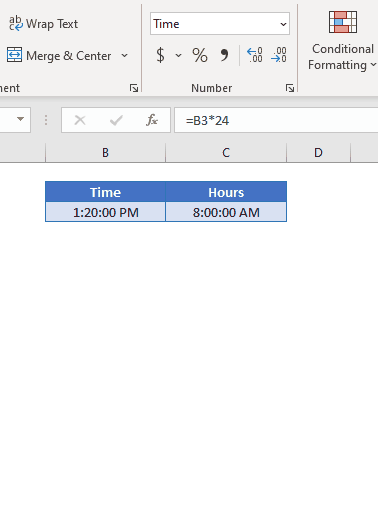## Convert Time to Hours in Google Sheets

All of the above examples work exactly the same in Google Sheets as in Excel.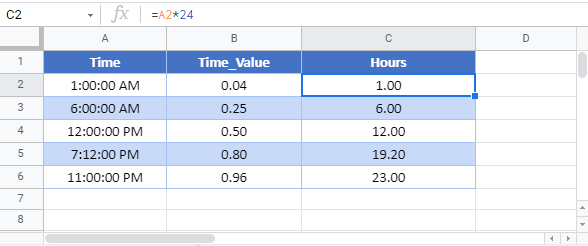### Excel Practice Worksheet

Practice Excel functions and formulas with our 100% free practice worksheets!